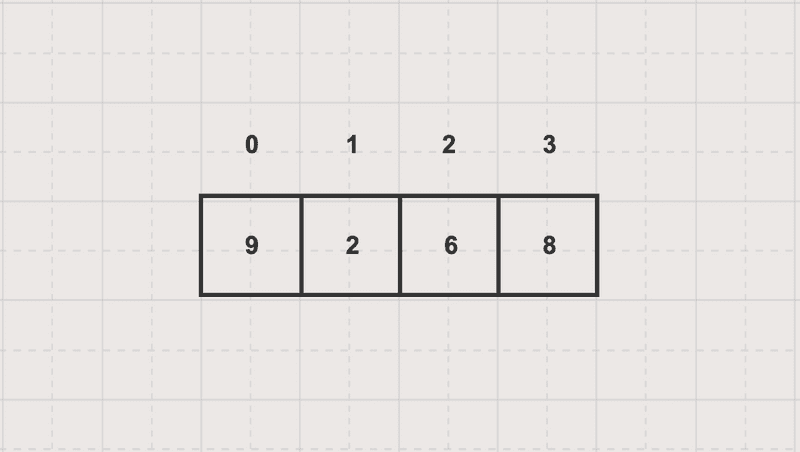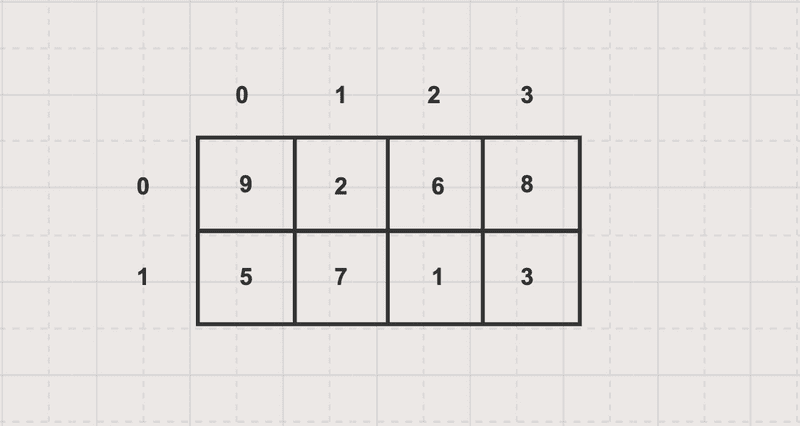# HelloKoding

Practical coding guides

# Array Data Structure

In this article, you will learn about one and two-dimensional Array data structures and theirs traversal examples

An array is a linear data structure consisting of a collection of elements which are stored in contiguous physical memory locations and identified by an index

## One-dimensional array

Given A as a one-dimensional array which has 4 elements like the below imageEach element in the array can be accessed directly by A, A, A and A with the corresponding value 9, 2, 6 and 8

Array1D.java

``````package com.hellokoding.datastructure;

public class Array1D {
void traversal(int[] A) {
for (int i = 0; i < A.length; i++) {
System.out.println(A[i]);
}
}

public static void main(String[] args) {
int[] A = {9, 2, 6, 8};
new Array1D().traversal(A);
}
}
``````

## Two-dimensional array

Given A as a two-dimensional array which has 8 elements like the below imageEach element in the array can be accessed directly by A, A, A, A, A, A, A and A with the corresponding value 9, 2, 6, 8, 5, 7, 1 and 3

Array2D.java

``````package com.hellokoding.datastructure;

public class Array2D {
void traversal(int[][] A) {
for (int i = 0; i < A.length; i++) {
for (int j = 0; j < A.length; j++) {
System.out.println(A[i][j]);
}
}
}

public static void main(String[] args) {
int[][] A = {{9, 2, 6, 8}, {5, 7, 1, 3}};
new Array2D().traversal(A);
}
}
``````